# 基于matlab的人脸识别系统设计与仿真

## 1.2 人脸图像识别的应用前景

 应用 优点 存在问题 信信用卡、汽车驾照、护照以及个人身份验证等 图像摄取可控图像分割可控图像质量好 需要建立庞大的数据库 嫌疑犯照片匹配 图像质量不统一多幅图像可用 潜在的巨大图像库 互联网应用 视频信息价值高多人参与 存在虚假 银行/储蓄安全 监控效果好 图像分割不可控图像质量较差 人群监测 图像质量高可利用摄像图像 图像分割自由图像质量低、实时性

(1)人脸图像的获取

(2)人脸的检测

(3)特征提取

(4)基于人脸图像比对的身份识别

(5)基于人脸图像比对的身份验证

## 2.2 数字图像处理及过程

### 2.2.2图像类型的转换

Matlab支持多种图像类型,但在某些图像操作中,对图像的类型有要求,所以要涉及到对图像类型进行转换。Matlab7.0图像处理工具箱为我们提供了不同图像类型相互转换的大量函数,如mat2gray()函数可以将矩阵转换为灰度图像,rgb2gray()转换RGB图像或颜色映像表为灰度图像。在类型转换的时候,我们还经常遇到数据类型不匹配的情况,针对这种情况,Matlab7.0工具箱中,也给我们提供了各种数据类型之间的转换函数,如double()就是把数据转换为双精度类型的函数。

(1).灰度变换增强

(2).空域滤波增强

## 2.3图像处理功能的Matlab实现实例

1)图像类型的转换

imshow(j);imwrite(j,'f:\face1.tif')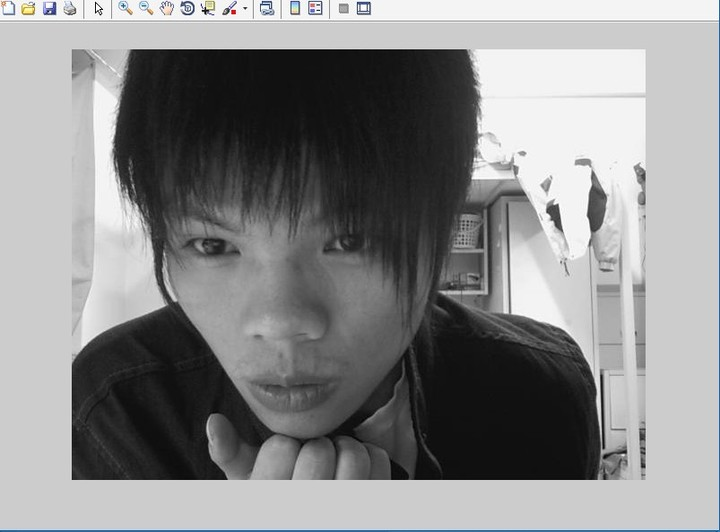2)图像增强

(1)灰度图像直方图均衡化

j=histeq(i);imshow(j);

figure,subplot(1,2,1),imhist(i);

subplot(1,2,2),imhist(j)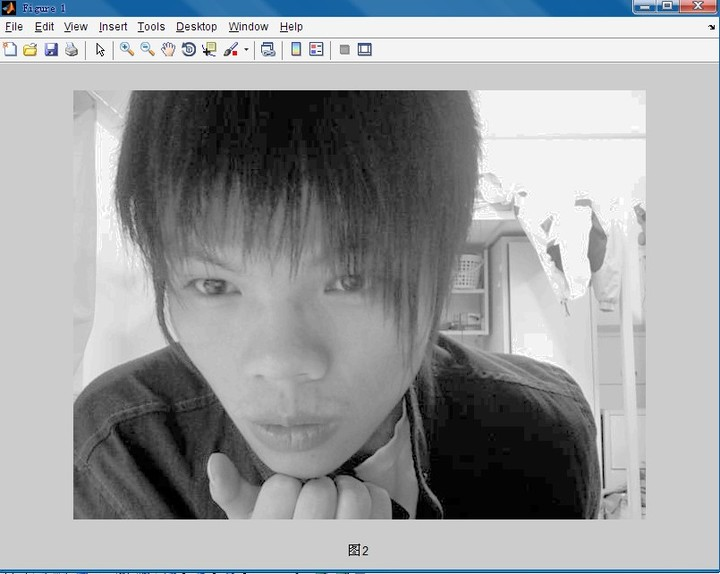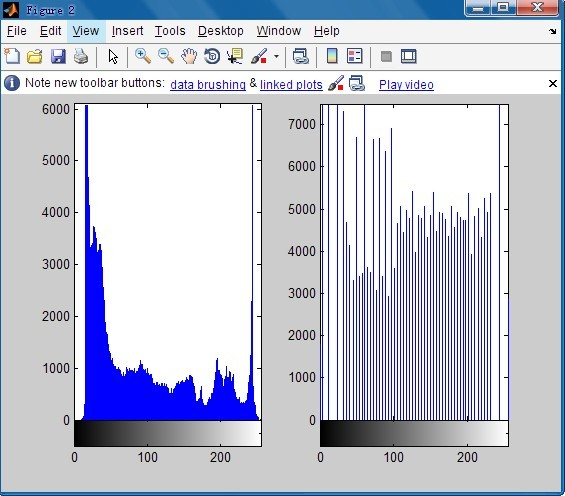(2)灰度图像平滑与锐化处理

j=imnoise(i,'guassian',0,0.02);

subplot(1,2,1),imshow(j);

j1=wiener2(j);subplot(1,2,2),imshow(j1);

h=fspecial('gaussian',2,0.05);j2=imfilter(i,h);figure,subplot(1,2,1),imshow(i)

subplot(1,2,2),imshow(j2)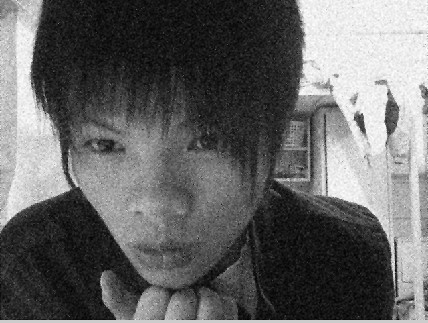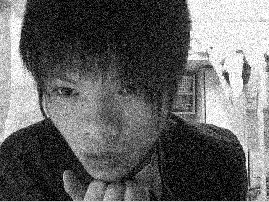3)边缘检测

Matlab7.0图像处理工具箱提供了edge()函数实现边缘检测,还

j=edge(i,'canny',[0.04,0.25],1.5);

imshow(j)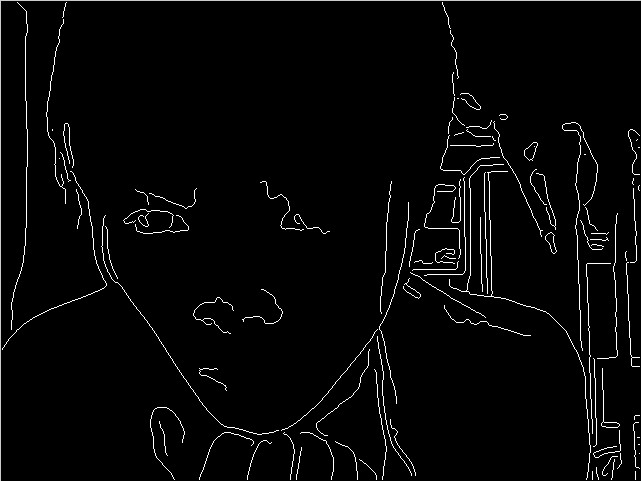## 3.2系统基本机构## 3.3 人脸检测定位算法

 检测方法 优点与适用场合 缺点与需要改进的地方 肤色模型 检测速度快 高光和阴影会造成人脸区域被分割而被漏检；肤色区域的存在提高了预警率 模板匹配 直观性好，具有较好的适应性 对表情、尺度变换敏感；可变模板的选择和参数的确定非常困难 基于知识的方法 适用复杂图像中的人脸检测 依赖先验知识；多尺度空间遍历工作量大，运算时间长

 检测方法 优点 缺点与需要改进的地方 本征脸法 标准人脸模板能抽象人脸全部信息，运算不涉及迭代耗费时间短 但模板检测效率低，多模板提高了效率也增加了检测时间 神经网络法 检测效率高，错误报警数目不多，训练成熟的网络监测速度快 多样本训练耗费时间多，但网络监测错误报警数目多 支撑向量机机法 比神经网络方法具有更好的泛化能力，能对为观测到的例子进行有效分类 “非人脸”样本复杂多样，造成支持向量数目多，运算复杂度大 基于积分图像分析法 检测速度快，基本满足实时检测要求，检测效率可以与神经网络法比较 错误报警数目少时，检测率不高

%%%%% Reading of a RGB image

I=rgb2gray(i);

BW=im2bw(I);

figure,imshow(BW)

%%%%%%%%%%%%%%%%%%%%%%%%%%%%%%%%%%%%%%%%%%%%%%%%%%%%%%

%%%%% minimisation of background portion

[n1 n2]=size(BW);

r=floor(n1/10);

c=floor(n2/10);

x1=1;x2=r;

s=r*c;

for i=1:10

y1=1;y2=c;

for j=1:10

if (y2<=c | y2>=9*c) | (x1==1 | x2==r*10)

loc=find(BW(x1:x2, y1:y2)==0);

[o p]=size(loc);

pr=o*100/s;

if pr<=100

BW(x1:x2, y1:y2)=0;

r1=x1;r2=x2;s1=y1;s2=y2;

pr1=0;

end

imshow(BW);

end

y1=y1+c;

y2=y2+c;

end

x1=x1+r;

x2=x2+r;

end

figure,imshow(BW)

%%%%%%%%%%%%%%%%%%%%%%%%%%%%%%%%%%%%%%%%%%%%%%%%

%%%%% detection of face object

L = bwlabel(BW,8);

BB = regionprops(L, 'BoundingBox');

BB1=struct2cell(BB);

BB2=cell2mat(BB1);

[s1 s2]=size(BB2);

mx=0;

for k=3:4:s2-1

p=BB2(1,k)*BB2(1,k+1);

if p>mx & (BB2(1,k)/BB2(1,k+1))<1.8

mx=p;

j=k;

end

end

figure,imshow(I);

hold on;

rectangle('Position',[BB2(1,j-2),BB2(1,j-1),BB2(1,j),BB2(1,j+1)],'EdgeColor','r' )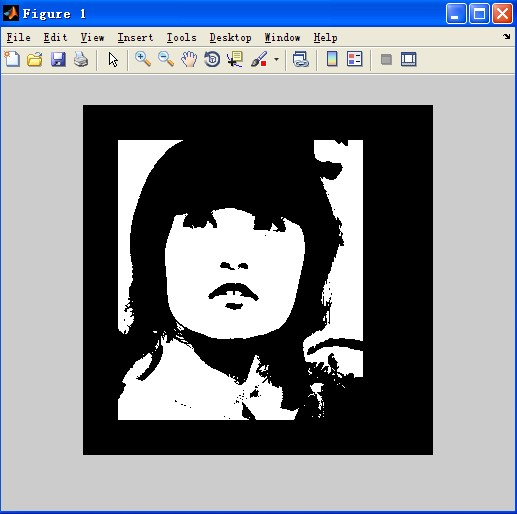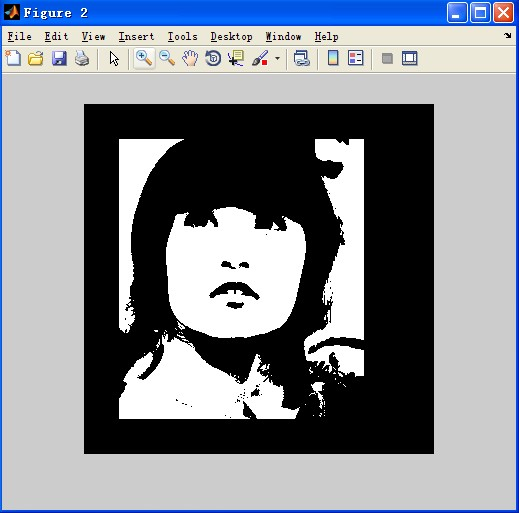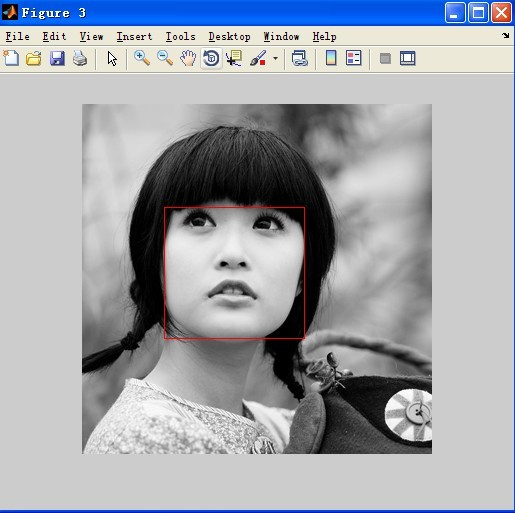## 3.4 人脸图像的预处理

### 3.4.1 仿真系统中实现的人脸图像预处理方法

1)滤波去噪

（1）线性滤波最典型的线性滤波方法如，采用邻域平均法的均值滤波器、采用邻域加权平均的高斯滤波和维纳滤波。对图像进行线性滤波可以去除图像中某些特定类型的噪声，如图像中的颗粒噪声，高斯噪声、椒盐噪声等。对扫描得到的人脸图像根据其噪声类型一般采用此种滤波方法。考虑滤波模板大小对滤波效果影响较大，仿真系统选择算法时对同种滤波算法提供了不同模板大小的情况。

（2）中值滤波法中值滤波法是一种非线性滤波方法，它把像素及其邻域中的像素按灰度级进行排序，然后选择该组的中间值作为输出像素值。中值滤波方法的最大优点是抑制噪声效果明显且能保护边界。对于使用基于整体的人脸识别算法的系统中，由于失掉小区域的细节对特征提取影响较小，所以这种滤波方法最受欢迎。

（3）自适应滤波自适应滤波能够根据图像的局部方差来调整滤波器的输出，其滤波效果要优于线性滤波，同时可以更好地保存图像的边缘和高频细节信息。

2)灰度变换

3)边缘检测

## 4.2 人脸识别的matlab实现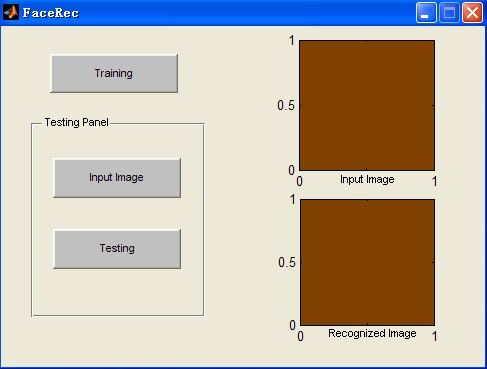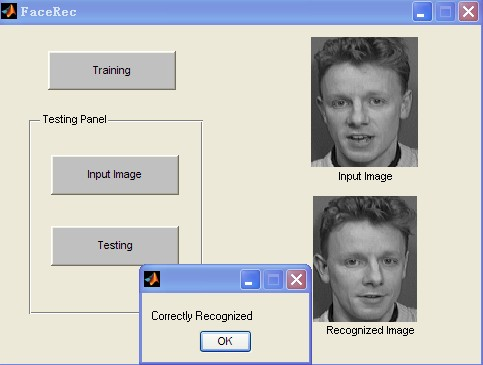## 参考文献

 祝磊，朱善安.人脸识别的一种新的特征提取方法[J].计算机学报，2007，34（6）：122-1251

 何东风，凌捷.人脸识别技术综述[J].计算机学报，2003，13（12）75-78

 ,Younus Fazl-e-Basit Javed和Usman Qayyum”,采用直方图的人脸识别和处理”,第三阶段仅相关新兴技术研报告。

 何国辉，甘俊英.PCA-LDA算法在性别鉴别中的应用[J].中国图像图形学报，2006，32（19）：208-211.

 王聃，贾云伟，林福严.人脸识别系统中的特征提取[J].自动化学报，2005，21（7-3）.

 张俭鸽，王世卿，盛光磊.基于小波和DFB-PCA的人脸识别算法研究[J].自动化学报，2007，23（2-1）.

 曹林，王东峰，刘小军，邹谋炎.基于二维Gabor小波的人脸识别算法[J].电子学报，2006，28（3）490-494

 焦峰，山世光，崔国勤，高文，李锦涛.基于局部特征分析的人脸识别方法[J].自动化学报，2003，15（1）：53-58

 Wangmeng Zuo，Kuanquan Wang，David Zhang，Hongzhi Zhang. Combination of two novel LDA-based methods for face recognition[C].Proceedings of the IEEE，2007：735-742

 徐倩，邓伟.一种融合两种主成分分析的人脸识别方法[J].计算机学报，2007，43（25）：195-197

function varargout = FR_Processed_histogram(varargin)
gui_Singleton = 1;
gui_State = struct('gui_Name',       mfilename, ...
'gui_Singleton',  gui_Singleton, ...
'gui_OpeningFcn', @FR_Processed_histogram_OpeningFcn, ...
'gui_OutputFcn',  @FR_Processed_histogram_OutputFcn, ...
'gui_LayoutFcn',  [] , ...
'gui_Callback',   []);
if nargin && ischar(varargin{1})
gui_State.gui_Callback = str2func(varargin{1});
end

if nargout
[varargout{1:nargout}] = gui_mainfcn(gui_State, varargin{:});
else
gui_mainfcn(gui_State, varargin{:});
end
% End initialization code - DO NOT EDIT

%--------------------------------------------------------------------------
% --- Executes just before FR_Processed_histogram is made visible.
function FR_Processed_histogram_OpeningFcn(hObject, eventdata, handles, varargin)

handles.output = hObject;

% Update handles structure
guidata(hObject, handles);

% UIWAIT makes FR_Processed_histogram wait for user response (see UIRESUME)
% uiwait(handles.figure1);
global total_sub train_img sub_img max_hist_level bin_num form_bin_num;

total_sub = 40;
train_img = 200;
sub_img = 10;
max_hist_level = 256;
bin_num = 9;
form_bin_num = 29;
%--------------------------------------------------------------------------
% --- Outputs from this function are returned to the command line.
function varargout = FR_Processed_histogram_OutputFcn(hObject, eventdata, handles)
varargout{1} = handles.output;

%--------------------------------------------------------------------------
% --- Executes on button press in train_button.
function train_button_Callback(hObject, eventdata, handles)
global train_processed_bin;
global total_sub train_img sub_img max_hist_level bin_num form_bin_num;

train_processed_bin(form_bin_num,train_img) = 0;
K = 1;
train_hist_img = zeros(max_hist_level, train_img);

for Z=1:1:total_sub
for X=1:2:sub_img    %%%train on odd number of images of each subject

[rows cols] = size(I);

for i=1:1:rows
for j=1:1:cols
if( I(i,j) == 0 )
train_hist_img(max_hist_level, K) =  train_hist_img(max_hist_level, K) + 1;
else
train_hist_img(I(i,j), K) = train_hist_img(I(i,j), K) + 1;
end
end
end
K = K + 1;
end
end

[r c] = size(train_hist_img);
sum = 0;
for i=1:1:c
K = 1;
for j=1:1:r
if( (mod(j,bin_num)) == 0 )
sum = sum + train_hist_img(j,i);
train_processed_bin(K,i) = sum/bin_num;
K = K + 1;
sum = 0;
else
sum = sum + train_hist_img(j,i);
end
end
train_processed_bin(K,i) = sum/bin_num;
end

display ('Training Done')
save 'train'  train_processed_bin;

%--------------------------------------------------------------------------
% --- Executes on button press in Testing_button.
function Testing_button_Callback(hObject, eventdata, handles)
global train_img max_hist_level bin_num form_bin_num;
global train_processed_bin;
global filename pathname I

test_hist_img(max_hist_level) = 0;
test_processed_bin(form_bin_num) = 0;

[rows cols] = size(I);

for i=1:1:rows
for j=1:1:cols
if( I(i,j) == 0 )
test_hist_img(max_hist_level) =  test_hist_img(max_hist_level) + 1;
else
test_hist_img(I(i,j)) = test_hist_img(I(i,j)) + 1;
end
end
end

[r c] = size(test_hist_img);
sum = 0;

K = 1;
for j=1:1:c
if( (mod(j,bin_num)) == 0 )
sum = sum + test_hist_img(j);
test_processed_bin(K) = sum/bin_num;
K = K + 1;
sum = 0;
else
sum = sum + test_hist_img(j);
end
end

test_processed_bin(K) = sum/bin_num;

sum = 0;
K = 1;

for y=1:1:train_img
for z=1:1:form_bin_num
sum = sum + abs( test_processed_bin(z) - train_processed_bin(z,y) );
end
img_bin_hist_sum(K,1) = sum;
sum = 0;
K = K + 1;
end

[temp M] = min(img_bin_hist_sum);
M = ceil(M/5);
getString_start=strfind(pathname,'S');
getString_start=getString_start(end)+1;
getString_end=strfind(pathname,'\');
getString_end=getString_end(end)-1;
subjectindex=str2num(pathname(getString_start:getString_end));

if (subjectindex == M)
axes (handles.axes3)
%image no: 5 is shown for visualization purpose
msgbox ( 'Correctly Recognized');
else
display ([ 'Error==>  Testing Image of Subject >>' num2str(subjectindex) '  matches with the image of subject >> '  num2str(M)])
axes (handles.axes3)
%image no: 5 is shown for visualization purpose
msgbox ( 'Incorrectly Recognized');
end

display('Testing Done')
%--------------------------------------------------------------------------
function box_Callback(hObject, eventdata, handles)
function box_CreateFcn(hObject, eventdata, handles)
if ispc && isequal(get(hObject,'BackgroundColor'), get(0,'defaultUicontrolBackgroundColor'))
set(hObject,'BackgroundColor','white');
end
%--------------------------------------------------------------------------
% --- Executes on button press in Input_Image_button.
function Input_Image_button_Callback(hObject, eventdata, handles)
% hObject    handle to Input_Image_button (see GCBO)
% eventdata  reserved - to be defined in a future version of MATLAB
% handles    structure with handles and user data (see GUIDATA)
global filename pathname I
[filename, pathname] = uigetfile('*.bmp', 'Test Image');
axes(handles.axes1)
imgpath=STRCAT(pathname,filename);

imshow(I)

%--------------------------------------------------------------------------
% --- Executes during object creation, after setting all properties.
function axes3_CreateFcn(hObject, eventdata, handles)

03-06

05-151万+
11-112万+
08-18335
08-291732
08-182208
11-014万+
03-202124
04-13129
02-134169
12-071522
03-132515
08-302199
10-132212
01-215397

### “相关推荐”对你有帮助么？

•非常没帮助
•没帮助
•一般
•有帮助
•非常有帮助MATLAB管家

¥2 ¥4 ¥6 ¥10 ¥20余额支付 (余额：-- )扫码支付获取中扫码支付点击重新获取扫码支付1.余额是钱包充值的虚拟货币，按照1:1的比例进行支付金额的抵扣。
2.余额无法直接购买下载，可以购买VIP、C币套餐、付费专栏及课程。余额充值